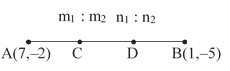Filter By

## All Questions

#### The points A (–1, –2), B (4, 3), C (2, 5) and D (–3, 0) in that order form a rectangle.

Solution.   The given points are A(–1, –2), B(4, 3), C(2, 5) and D(–3, 0)

AB = CD , BC = DA

AC = BD (Diagonals)
Hence, ABCD is a rectangle because
AB = CD, BC = DA, AC = BD

#### The point P (–2, 4) lies on a circle of radius 6 and centre C (3, 5).

Solution.
The radius of the circle is 6 and centre C(3, 5)
If point P(–2, 4) lies on the circle then the distance between the centre and point P is equal to the radius of the circle.

(x1, y1) = (-2, 4)             (x2, y2) = (3, 5)

Hence, point P(–2, 4) not lies on the circle with centre C(3, 5).

## Crack CUET with india's "Best Teachers"

• HD Video Lectures
• Unlimited Mock Tests
• Faculty Support#### Points A (–6, 10), B (–4, 6) and C (3, –8) are collinear such that AB =2/9 AC.

Solution.     If the points A (–6, 10), B(–4, 6) and C(3, –8) are collinear then area of ABC = 0

Area of ABC = 0
Hence, A, B and C are collinear

Hence,

#### Point P (5, –3) is one of the two points of trisection of the line segment joining the points A (7, – 2) and B (1, – 5).

Solution.Let the two point of trisection are C, D

(x1, y1) = (7, -2)           (x2, y2) = (1, -5)
m1 = 1 , m2 = 2    (Because C divide AB in ratio 1:2 )

n1 = 2 , n2 = 1    (Because D divide AB in ratio 2:1)

Hence, the given statement is true.

## Crack NEET with "AI Coach"

• HD Video Lectures
• Unlimited Mock Tests
• Faculty Support#### The point A (2, 7) lies on the perpendicular bisector of line segment joining the points P (6, 5) and Q (0, – 4).

Solution.     If point A(2, 7) is bisector then it must be mid-point of the line joining the points P(6, 5) and Q(0, –4)

(x1, y1) = (6, 5)        (x2, y2) = (0, -4)

Hence, A not lies on bisector.

#### A circle has its Centre at the origin and a point P (5, 0) lies on it. The point Q (6, 8) lies outside the circle.

Solution.    The centre of the circle is O (0, 0).
If point P(5, 0) lies on the circle then the distance between O(0, 0) and P(5, 0) is the radius of the circle

OP = 5
If point Q (6, 8) is outside the circle then the distance between O(0, 0) and Q(6, 8) is grater then the radius of the circle
(x1, y1) = (0, 0)                        (x2, y2) = (6, 8)

Here point OQ is greater than the radius of circle
Hence, point Q(6, 8) lies outside the circle

## Crack JEE Main with "AI Coach"

• HD Video Lectures
• Unlimited Mock Tests
• Faculty Support#### Points A (4, 3), B (6, 4), C (5, –6) and D (–3, 5) are the vertices of a parallelogram.

Solution.
The given points area A(4, 3), B(6, 4), C(5, –6), D(–3, 5)

Here, opposite sides are not equal i.e.

Hence, it is not a parallelogram

#### Points A (3, 1), B(12, –2) and C(0, 2) cannot be the vertices of a triangle.

Solution.         If they are not the vertices of a triangle then
Area of ABC = 0
x1 =3    x2 =12     x3 =0
y1=1     y2=-2     y3=2
Let us find the area of ABC

=   0

Area of ABC = 0
Hence, they are collinear or not the vertices of a triangle.

## Crack CUET with india's "Best Teachers"

• HD Video Lectures
• Unlimited Mock Tests
• Faculty Support#### Point P (0, 2) is the point of intersection of y–axis and perpendicular bisector of line segment joining the points A (–1, 1) and B (3, 3).

Solution.      If the point P is a perpendicular bisector of the line joining the point A(–1, 1) and B(3, 3) then it must be mid-point of AB.

(x1, y1) = (-1, 1)        (x2, y2) = (3, 3)

Which is not point P.
Hence, the given statement is false.

#### The points (0, 5), (0, –9) and (3, 6) are collinear.

The given points area (0, 5), (0, –9) and (3, 6)
If the point area collinear then the area of triangle is 0.
x1 =0    x2 =0     x3 =3
y1=5     y2=9     y3=6

Area of triangle = 21
Here the area of the triangle is not equal to zero.
Hence, the point is not collinear.